# 2019 HSC Chemistry Exam Paper Worked Solutions

In this post, our Science Team reveals the answers to the 2019 HSC Chemistry Exam paper.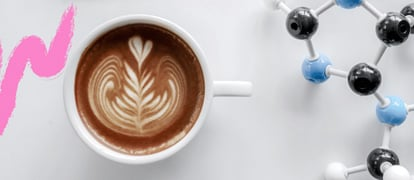Keen to see how you went in the 2019 HSC Chemistry exam? Well, our Chemistry team have been hard at work cooking up the solutions for you!

## 2019 HSC Chemistry Exam Paper Worked Solutions

Below are the answers and worked solutions to this year’s Chemistry HSC Exam.

## Section 1: Multiple Choice Solutions

 Question Solution Explanation 1 D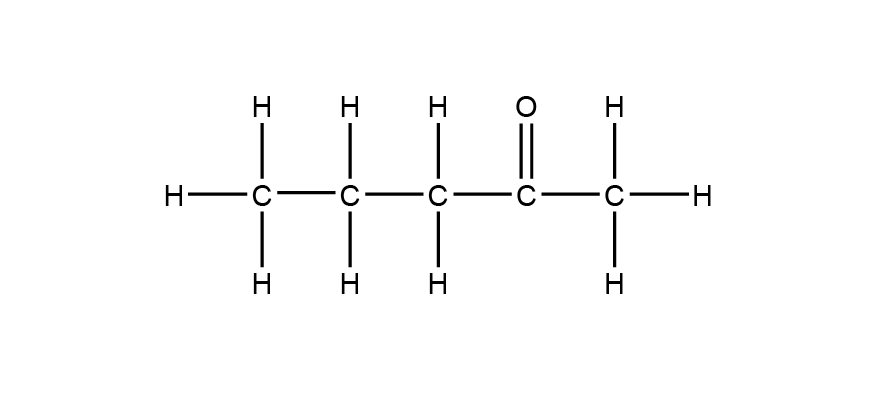2 B An Arrhenius base is a substance that donates OH– when dissolved in water. 3 A Calcium carbonate has the highest Ksp value of the given metal carbonates. As each of the metal carbonates produce the same number of ions in solution, their values can be directly compared to determine the substance with the highest molar solubility. 4 B The mass to charge ratio (m/z) of the parent ion (molecular ion) is 88. This corresponds to the molecular mass of butanoic acid.Note: the other fragments in the mass spectrum are due to a complex rearrangement reaction followed by fragmentation process (McLafferty rearrangement). As a result, the m/z values of the other fragments do not correspond to the fragments that would be anticipated for the butanoic acid molecule. 5 C The colour change range of isopicramic acid (pH 4.0–5.6) contains the equivalence point of the titration (approximately pH 5). 6 A The titration curve is of a weak base NH3 being titrated by a strong acid HCl. 7 D A catalyst provides an alternative reaction pathway for a reaction that has a lower activation energy. The reduction in activation energy is the same for both forward and reverse reactions. 8 A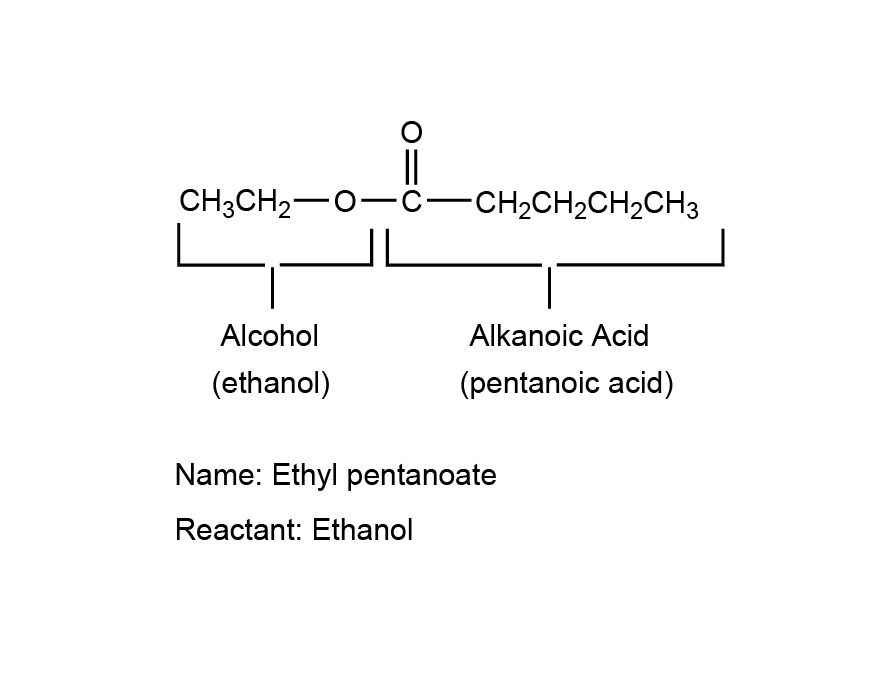9 B Hydrogen bonding is the strongest intermolecular force. Carboxylic acids can form the greatest number of hydrogen bonding interactions, thus requiring more thermal energy to overcome the intermolecular forces when going from the liquid to the gas state (boiling). 10 D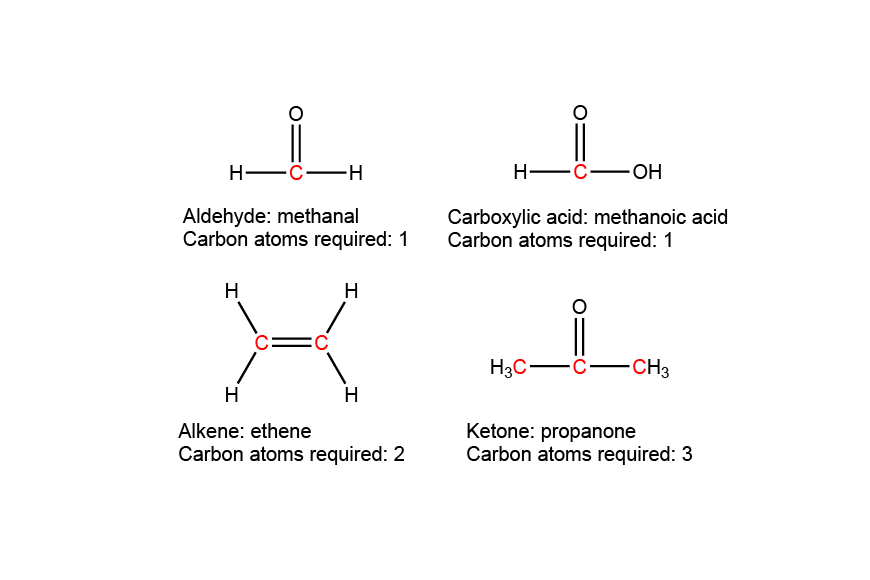11 C In a saturated solution of barium carbonate, the solid and aqueous barium carbonate are in dynamic equilibrium:BaCO3(s) ⇌ Ba2+(aq) + CO32-(aq)Even though there will be no macroscopic signs of change, the forward and reverse reactions are still proceeding. Therefore, solid barium carbonate containing radioactive carbon-14 will dissolve into the solution. After some time, the radioactive isotope will be present in both the solid residue and the filtrate solution. 12 C To maximise yield, the equilibrium must shift in the forward direction.High pressure: The forward reaction converts 3 moles of gas to 2 moles of gas. According to Le Chatelier’s principle, when pressure is increased, the equilibrium shifts to the side with less gas moles to reduce pressure and minimise the disturbance.Low temperature: The forward reaction is exothermic. According to Le Chatelier’s principle, when temperature is decreased, the equilibrium shifts to the exothermic side to replace heat and minimise the disturbance. 13 B The repeating unit of the polymer is C2F2H2The molar mass of the repeating unit is 64.036 g mol-1The number of repeating units is (4.8 x 104) / 64.036 = 750There are two carbons per repeating unit, so number of carbons is 750 x 2 = 1500 14 A There is only one signal in the 1H NMR spectrum, indicating there is only one hydrogen environment. This means all the hydrogens must be in the same environment.In A, all the hydrogens are equivalent.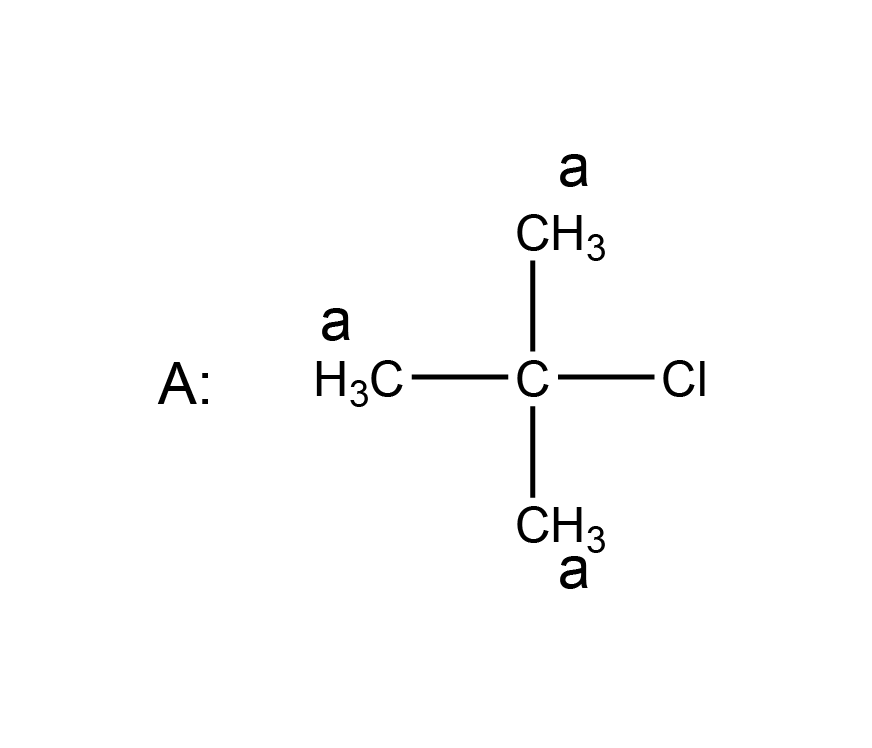B is incorrect as there are three hydrogen environments and so 3 signals are expected. C and D are incorrect as there are four hydrogen environments, so four signals are expected.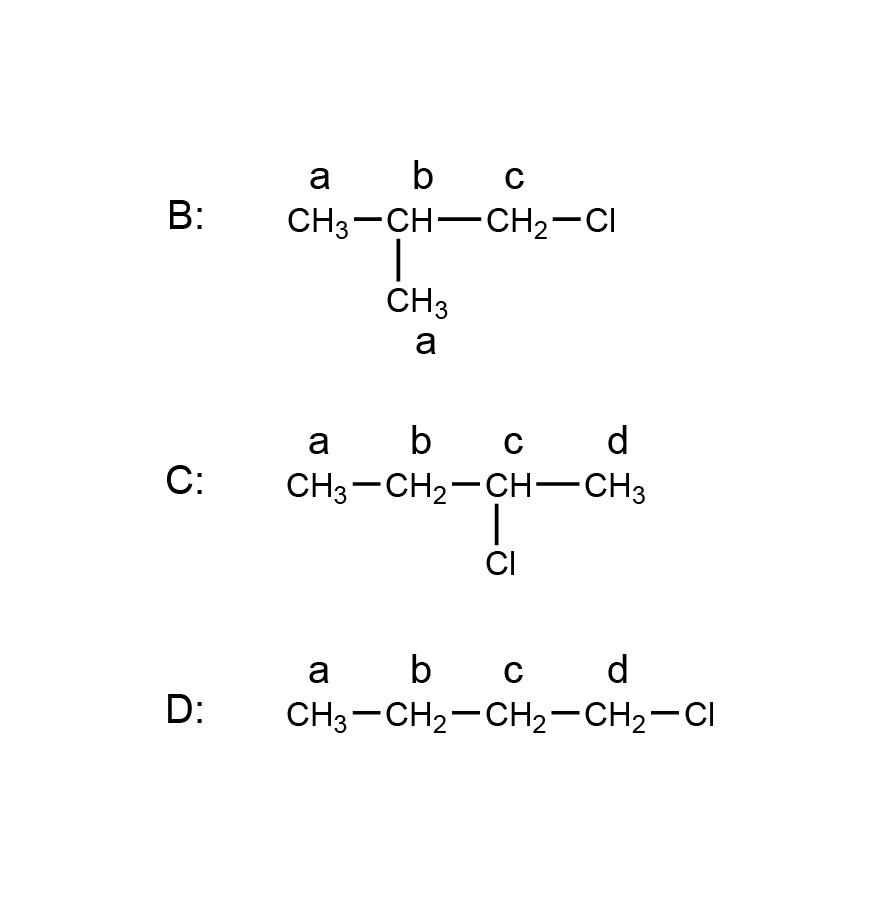15 B pOH = 14 – 8.53 = 5.47[OH-] = 10-5.47 = 3.4 x 10-6 16 B $$K_{eq} = \frac{[HI]^2}{[H_2][I_2]} = \frac{0.358^2}{0.0430 \times 0.0620} = 48.1$$ 17 D Precipitation reaction:Ba(NO3)2(aq)  +  2NaOH(aq)  →  Ba(OH)2(s)  + 2NaNO3(aq)  Precipitate that could be formed is barium hydroxide.Ba(OH)2(s)   ⇌   Ba2+(aq)   +   2OH–(aq)Ksp = [Ba2+][OH–]2 = 2.55 x 10-4Equal volumes of barium nitrate and sodium hydroxide means concentrations will be halved.[Ba2+] = 0.025 mol L-1[OH-] = 0.05 mol L-1Q = (0.025)(0.05)2 = 6.25 x 10-5Since Q < Ksp, no precipitate will form. 18 A Keq is very small, thus at equilibrium there is a greater concentration of reactants than products and the equilibrium lies to the left. This means the reverse reaction is favoured and F– preferentially accepts a proton compared to CF3COO–, making it a stronger base. Similarly, CF3COOH will preferentially donate a proton compared to HF, making it the stronger acid. 19 D Acidified potassium permanganate is an oxidising agent so compound Y is produced from the oxidation of X. Compound Y turns blue litmus red. Thus, compound Y must be a carboxylic acid and compound X must be a primary alcohol. Treatment of compound X (an alcohol) with hot concentrated sulfuric acid results in a dehydration reaction.A is incorrect because butan-1-ol would produce 4 signals in the 13C NMR spectrum.B is incorrect because butan-2-ol is not a primary alcohol.C is incorrect because methyl ethanoate cannot be oxidised to methanoic acid. 20 C Concentration of MnO4– at absorbance 0.5 = 25 mg L-1 (from graph) $$c (\textrm{dilute} \thinspace MnO_4^-) = \frac{25 \times 10^{-3}}{54.94 + 16 \times 4}= 2.1019 \times 10^{-4} \textrm{ mol L}^{-1}$$ $$c( \textrm{concentrated} \thinspace MnO_4^-) = \frac{2.1019 \times 10^{-4} \times 0.100}{0.200}=1.05095 \times 10^{-3} \textrm{ mol L}^{-1}$$ Therefore, in 1 L: $$n(MnO_4^-) = 1.05095 \times 10^{-3} \textrm{ mol}$$ $$n(Mn^{2+}) = n(MnO_{4}^-) = 1.05095 \times 10^{-3} \textrm{ mol}$$ $$m(Mn^{2+}) = n \times MM = 1.05095 \times 10^{-3} \times 54.94 = 5.7739 \times 10^{-3} \thinspace g$$$$m(Mn^{2+})/m(\textrm{sample}) \times 100 = \frac{5.7739 \times 10^{-3}}{12.0} \times 100 = 0.48\%$$

## Section 2: Long response Questions

### Question 21a (2 marks)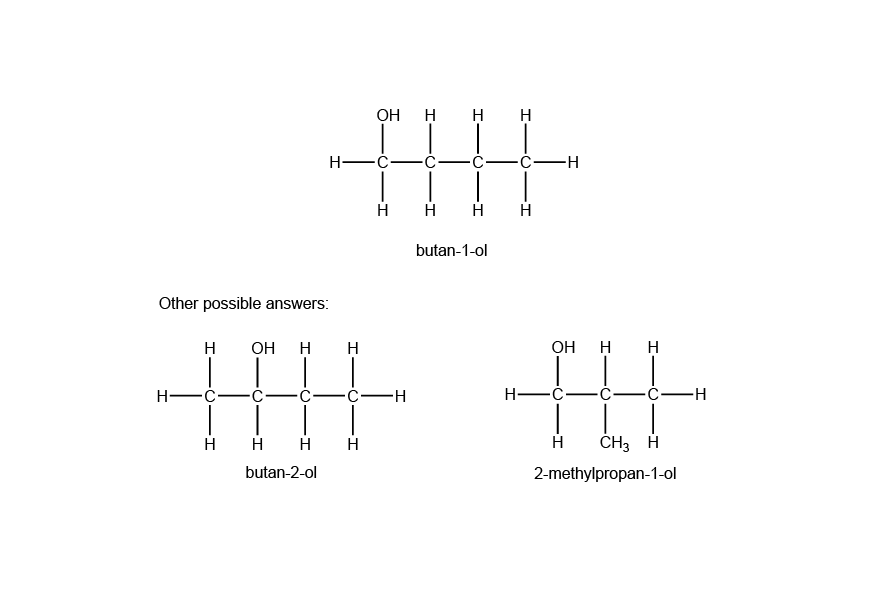### Question 21b (2 marks)

The two compounds have the same molecular formula of C3H6O, however, the atoms in the molecules are arranged in different ways such that they have different functional groups. Isomer A contains a ketone functional group whereas isomer B contains an aldehyde functional group.

### Question 21c (3 marks)

Isomer A can be distinguished from isomer B by their reaction with acidified potassium permanganate. Isomer B can be oxidised to propanoic acid. The reaction is indicated by a colour change from purple to colourless. However, isomer A will not cause a colour change as it cannot be further oxidised.

### Question 22 (4 marks)

A buffer is a solution which resists changes in pH when small quantities of acid or base are added. A solution containing CH3COOH and CH3COO (from NaCH3COO) will exist in equilibrium as follows:
CH3COOH(aq) + H2O(l) ⇌ CH3COO(aq) + H3O+(aq)

When a small amount of 0.1 mol L-1 HCl is added to the buffer solution, [H3O+] increases, disturbing the equilibrium. According to Le Chatelier’s principle, equilibrium shifts to the left to use up H3O+ and minimise the disturbance. Hence [H3O+] remains relatively stable, and since pH = -log10[H3O+], pH remains relatively stable as well. Similarly, when 0.1 mol L-1 NaOH is added, the OH ions will react with H3O+ ions, reducing their concentration in the equilibrium mixture. This will shift the equilibrium to the right according to Le Chatelier’s principle, increasing the H3O+ concentration to minimise the disturbance.

Since the pH remains relatively stable when HCl and NaOH are added, there will be no change in the colour of the indicator.

### Question 23a (4 marks)

q(H2O) = mc∆T = 105 x 10-3 x 4.18 x 103 x (30 – 18.5) = 5047.35 J = 5.04735 kJ

n(C2H5OH) = m / MM = 0.370 / 46.068 = 8.0316 x 10-3 mol

∆H = -q / n = -5.04735 / 8.0316 x 10-3 = -628 kJ mol-1 (3 s.f.)

### Question 23b (2 marks)

The spirit burner can be brought close to the beaker of water. This will minimise the heat loss to the surroundings. Since more of the heat produced from the combustion reaction will be absorbed by the water, the calculated ∆H value will be greater in magnitude and thus closer to the accepted value.

(Other possible answers: Use clamp and retort stand instead of tripod and gauze mat or insulate the calorimeter with heat shields)

### Question 24a (3 marks)

The conductivity initially is very high because of a high concentration of ions in solution, which results from the complete ionisation of hydrochloric acid. As barium hydroxide is added, the conductivity decreases because the concentration of the ions decreases since the moles of ions decrease as neutralisation occurs and the volume is increased. Additionally, as neutralisation occurs, H+ ions are replaced by less conductive Ba2+ ions. The conductivity reaches a minimum at the equivalence point, as all the H+ ions are removed and only Ba2+ and Cl ions are present. After the equivalence point, conductivity increases as more barium hydroxide is added. Since no reaction occurs, the moles of ions increases in the solution. Therefore, the concentration of ions increases resulting in increasing conductivity.

### Question 24b (4 marks)

Ba(OH)2(aq) + 2HCl(aq) → BaCl2(aq) + 2H2O(l)
n(HCl) = c x V = 1.050 x 10-3 x 0.250 = 2.625 x 10-4 mol
n(Ba(OH)2) = ½ x n(HCl) = 1.3125 x 10-4 mol
c(Ba(OH)2) = n / V = 1.3125 x 10-4 / 0.01715 = 7.653 x 10-3 mol L-1 (4 s.f.)

### Question 25a (2 marks)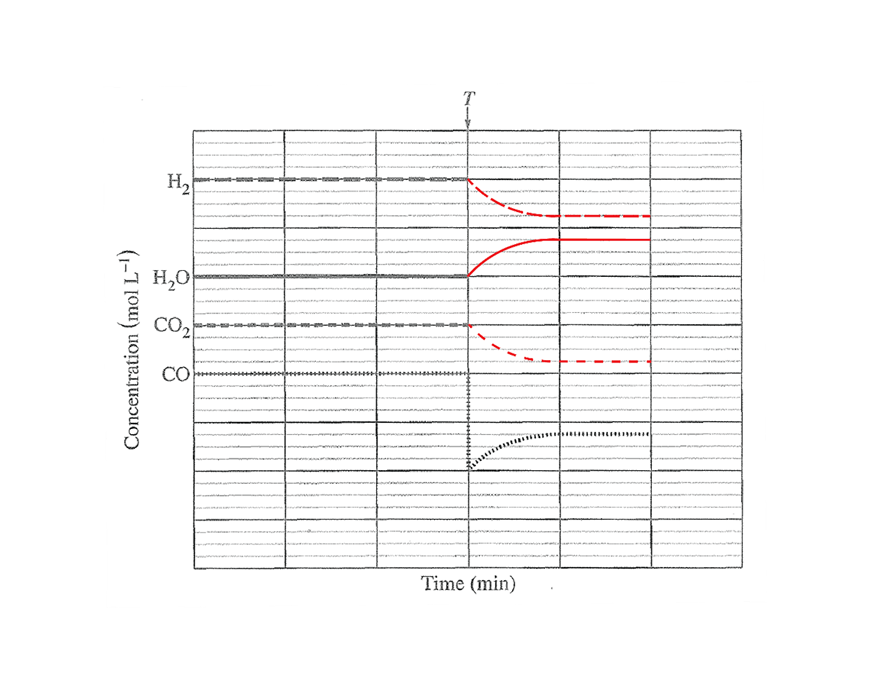### Question 25b (3 marks)

The removal of CO decreases its concentration, which causes a decrease in the frequency of successful collisions between CO and H2O. Hence the rate of the forward reaction decreases while the reverse rate remains unchanged as the concentrations of CO2 and H2 are unchanged. Since the rate of the forward reaction is slower than the reverse reaction, the equilibrium is shifted left, resulting in an increase in the concentration of CO. Over time, as more CO and H2O are formed and CO2 and H2 concentrations decrease, the rate of the forward reaction increases while the rate of the reverse reaction decreases until the rates become equal again and all the concentrations remain constant.

### Question 26a (5 marks)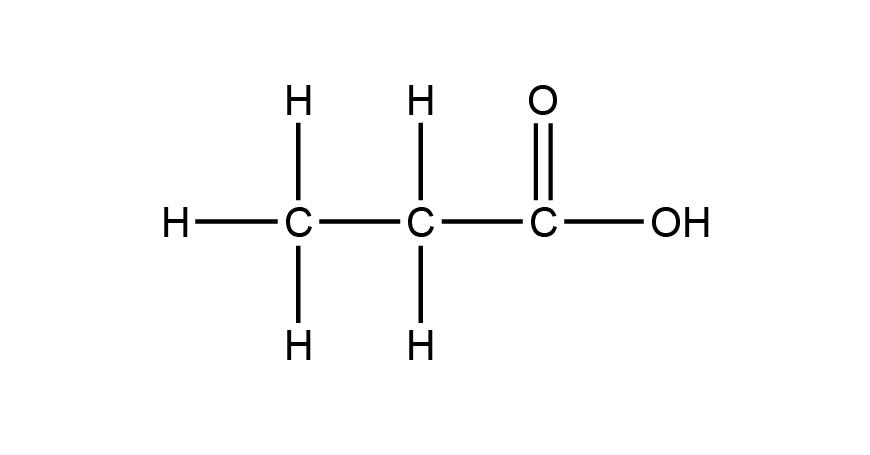The IR spectrum contains a strong absorption around 1700 cm-1 which is characteristic of a C=O bond and another broad absorption in the region 2500–3500 cm-1 which is characteristic of an O–H bond in acids.The compound reacts with sodium carbonate powder to produce carbon dioxide bubbles. This indicates the compound is a carboxylic acid.

The mass spectrum has a parent peak at m/z = 74, which is consistent with the molecular formula C3H6O2. The peaks at m/z = 45 and 57 correspond with the fragment ions: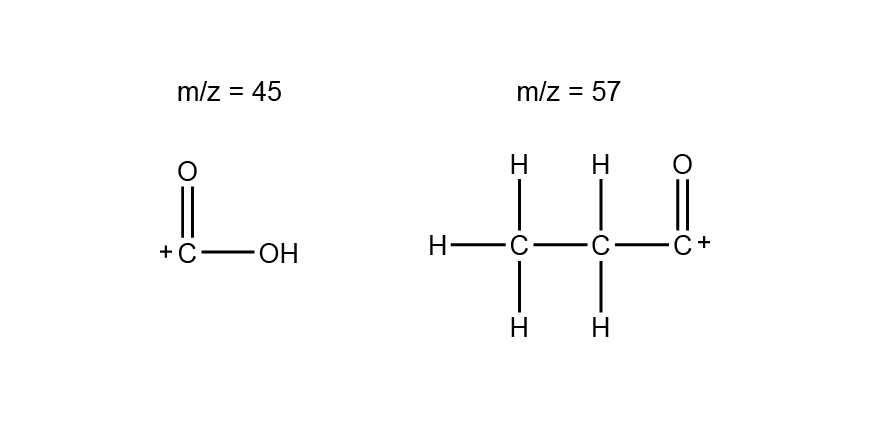The 13C NMR spectrum shows 3 signals, indicating that each carbon is in a different carbon environment. The signal around 180 ppm is characteristic of a carbonyl carbon.

Can also include:

The 1H NMR spectrum shows 3 signals:

• A singlet with an integration of 1 which indicates there are no neighbouring hydrogens and only one hydrogen in this environment. This corresponds to the acidic hydrogen.
• A quartet with an integration of 2 which indicates the presence of a neighbouring –CH3 and two hydrogens in this environment. This corresponds to the hydrogens on the second carbon.
• A triplet with an integration of 3 indicating the presence of a neighbouring –CH2– and three hydrogens in this environment. This corresponds to the hydrogens on the third carbon.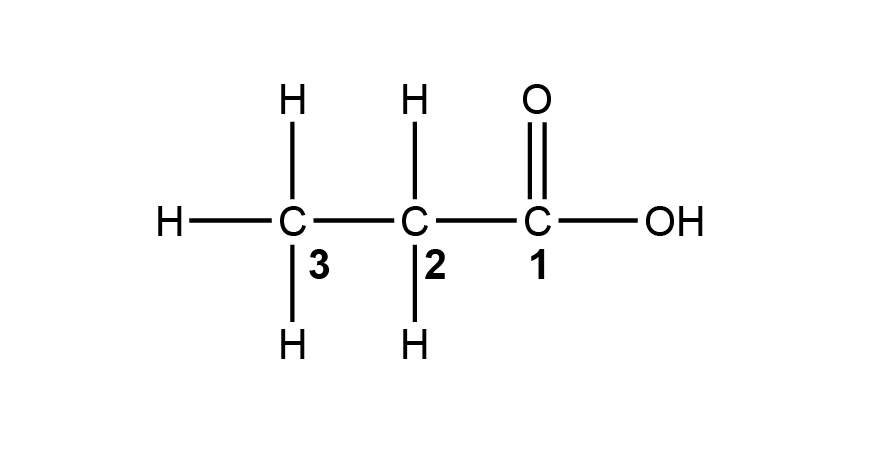### Question 26b (3 marks)

Chemists should use more than one spectroscopic technique because different techniques provide different pieces of information about the structure of an organic compound. For example, NMR spectroscopy gives information about the type of bond present, through the chemical shifts, and provides information about the connectivity, through the splitting pattern in the 1H NMR spectrum. IR spectroscopy gives information about the bonds or functional groups present.

The use of a combination of techniques allows complete elucidation of a structure and ensures the proposed structure is correct as it is supported by multiple sets of data.

### Question 27a (1 mark)

Kb = Kw / Ka = 10-14 / 3.0 x 10-8 = 3.3 x 10-7

### Question 27b (4 marks)

 Concentration (mol L-1) OCl– HOCl OH– Initial 0.20 0 0 Change -𝑥 +𝑥 +𝑥 Equilibrium 0.20 – 𝑥 𝑥 𝑥

Assume 0.20 – 𝑥 ≈ 0.20 since Kb is small.
Kb = 𝑥2 / 0.20 = 3.3 x 10-7
𝑥 = 2.569 x 10-4
Therefore [OH] = 2.569 x 10-4 mol L-1
pOH = -log10(2.569 x 10-4) = 3.59
pH = 14 – 3.59 = 10.41 (2 s.f.)

### Question 28

The Bronsted-Lowry model of classifying acids and bases defines an acid as a proton (H+) donor and a base as a proton (H+) acceptor. This model is better able to classify acids and bases than the Arrhenius model.

Unlike the Arrhenius definition of acids and bases, the Bronsted-Lowry model was able to explain the basic nature of species that do not contain OH. For example, the basic nature of ammonia (NH3):

NH3(aq) + H2O(I) ⇌ NH4+(aq) + OH(aq)

The Bronsted-Lowry model was also able to explain non-aqueous acid base reactions. For example, the reaction between hydrogen chloride and ammonia gases:

NH3(g) + HCI(g) → NH4Cl(s)

Consequently, the Bronsted-Lowry theory was an improvement on the previous theories of acids and bases as it explained the acid-base behaviour of more species.

### Question 29a (2 marks)

Addition of solid Ca(OH)2 to the stormwater produces the ions Ca2+ and OH. The Pb2+ and Cu2+ ions will form precipitates with the OH ions according to the following equations:

Pb2+(aq) + 2OH(aq) ⇌ Pb(OH)2(s)

Cu2+(aq) + 2OH(aq) ⇌ Cu(OH)2(s)

### Question 29b (2 marks)

In order to measure the concentration of an element in a sample, AAS uses a wavelength of light that corresponds to an atomic absorption of that element. As each element has unique wavelengths of light that are absorbed, the concentration of the analyte can be selectively measured in the presence of other species. Consequently, the concentrations of the Pb2+ and Cu2+ can be independently measured in a stormwater sample containing both ions.

### Question 29c (7 marks)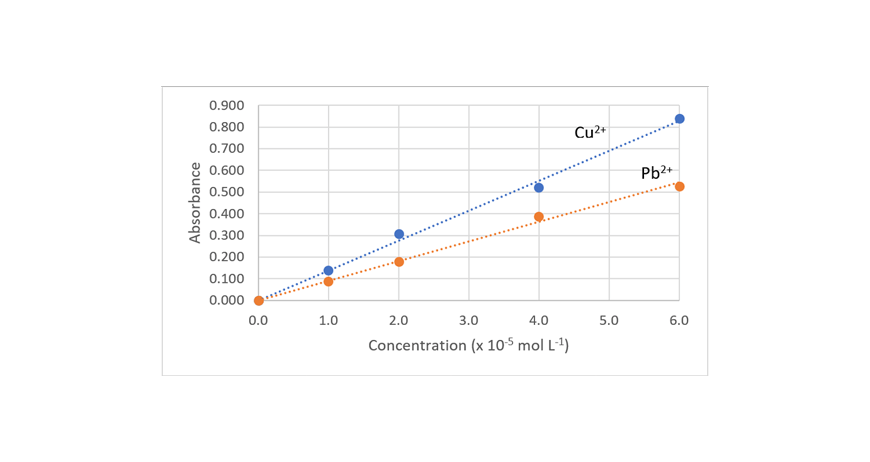Measured absorbance of Cu2+: 0.040 = 0.3 x 10-5 mol L-1 (from graph)
c(Cu2+ after treatment) = 0.3 x 10-5 mol L-1 x 63.55 g mol-1
= 1.9065 x 10-4 g L-1
= 0.19 mg L-1 (2 s.f.)

Measured absorbance of Pb2+: 0.080 = 0.9 x 10-5 mol L-1 (from graph)
c(Pb2+ after treatment) = 0.9 x 10-5 mol L-1 x 207 g mol-1
= 1.863 x 10-3 g L-1
= 1.9 mg L-1 (2 s.f.)

Therefore, the treatment is effective in reducing the concentration of the Cu2+ ions below the discharge limit, however is unable to reduce the concentration of Pb2+ below the discharge limit.

### Question 30 (3 marks)

For these salts to be soluble, ΔG must be less than zero (ΔG < 0). As a result, magnesium chloride is soluble in water at 298 K while magnesium fluoride is not.

Considering the equation, ΔG = ΔH – TΔS:

The dissolution of both magnesium chloride and magnesium fluoride are both exothermic (ΔH < 0). The enthalpy change associated with the dissolution of magnesium chloride is however more negative than that of magnesium fluoride, meaning it is more enthalpically favourable.

The dissolution of magnesium chloride and magnesium fluoride both have a negative entropy change (ΔS), making each process entropically unfavourable (for a process to be favourable, ΔS > 0). The entropy change for magnesium fluoride is however more negative than that of magnesium chloride.

To overcome the negative entropy change associated with the dissolution of each of these salts at 298 K (TΔS values), the process must have a large negative ΔH to be favourable. As a consequence, magnesium chloride is soluble, while magnesium fluoride is not.

### Question 31 (4 marks)

 Concentration (mol L-1) HgCl42- Cu2+ CuCl42- Hg2+ Initial 0.100 0.100 0 0 Change -𝑥 -𝑥 +𝑥 +𝑥 Equilibrium 0.100 – 𝑥 0.100 – 𝑥 𝑥 𝑥

Keq = 𝑥2 / (0.100 – 𝑥)2 = 4.55 x 10-11
Taking the square root of both sides:
𝑥 / (0.100 – 𝑥) = 6.74536878 x 10-6
𝑥 = 6.74536878 x 10-6 x 0.100 / (1 + 6.74536878 x 10-6) = 6.745323 x 10-7
[Hg2+] = 6.75 x 10-7 mol L-1 (3 s.f.)

### Question 32 (4 marks)

Considering the simplest examples of each class of compound, the dominant intermolecular force of methanol is hydrogen bonding while for methanethiol it is dipole-dipole forces. As hydrogen bonding is a stronger intermolecular force than dipole-dipole forces, less heat energy is required to overcome the intermolecular forces, meaning that the thiol compounds have lower boiling points than their alcohol analogues.

However, as the chain length increases, the difference in boiling point between the alcohols and thiols decreases. This is due to the increasing strength of the dispersion forces (dispersion forces are proportional to molecular mass). As the thiol compounds have a greater molecular mass than their alcohol counterparts, the dispersion forces become a more dominant intermolecular force in determining boiling point. This results in a more dramatic increase in their boiling points with chain length relative to the alcohols.

### Question 33 (4 marks)

Al(OH)3(aq) + 3HCl(aq) → AlCl3(aq) + 3H2O(l)
n(Al(OH)3) = m / MM = 1.17 / 78.004 = 0.014999 mol
n(HCl) = c x V = 0.100 x 0.500 = 0.05 mol
Al(OH)3 is limiting reactant and HCl is in excess
n(HCl) remaining = n(HCl) initial – n(HCl) used = 0.05 – 0.044998
= 5.002308 x 10-3 mol
n(H+) = n(HCl) = 5.002308 x 10-3 mol
[H+] = n / V = 5.002308 x 10-3 / 0.500 = 0.0100046 mol L-1
pH = -log10(0.0100046) = 2.000 (3 s.f.)

### Question 34 (7 marks)

Step 1: NaOH(aq), heat
Step 2: KMnO4/H+, heat
Step 3: Concentrated sulfuric acid, reflux

Product B can be identified using infrared spectroscopy. A broad absorption between 3230 cm-1 and 3550 cm-1 would indicate the presence of an O–H bond. This absorption would not be observed with reactant A.
Product C can be identified from its reaction with a solution of sodium carbonate. The presence of the carboxylic acid is confirmed by the production of carbon dioxide bubbles.
Product D can be identified by its insolubility in water. As product D cannot form hydrogen bonds with water, it will be poorly soluble. The reactants B and C would be soluble in water as they can form hydrogen bonds with water.

## Want to know your ATAR?

Use the calculator to explore the HSC Marks you need to achieve your ATAR Goal.### Get free study tips and resources delivered to your inbox.

Join 75,893 students who already have a head start.

Our website uses cookies to provide you with a better browsing experience. If you continue to use this site, you consent to our use of cookies. Read our cookies statement.